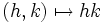# Topological factor

This article defines a property that can be evaluated for a subgroup of a semitopological group

## Contents

BEWARE! This term is nonstandard and is being used locally within the wiki. [SHOW MORE]

## Definition

A closed subgroup$H$ of a topological group$G$ is termed a topological factor if the following equivalent conditions are satisfied:

• The quotient bundle$p:G \to G/H$ is a trivial fiber bundle
• There exists a subset$K$ of$G$ (not necessarily a subgroup) such that the map$H \times K \to G$ given by$(h,k) \mapsto hk$, is a homeomorphism of topological spaces (this forces$K$ to intersect every right coset of$H$ at one point).

## Metaproperties

### Transitivity

This property of a subgroup in a topological group is transitive

A topological factor of a topological factor is a topological factor. This follows from the fact that a trivial bundle over a trivial bundle is a trivial bundle, or equivalently, from associativity of the product topology.

### Intermediate subgroup condition

This property satisfies the topological intermediate subgroup condition: in other words, if a subgroup satisfies the property in the whole group, it also satisfies the property in every intermediate subgroup

If$H$ is a topological factor of$G$, and$M$ is any intermediate subgroup, then$H$ is also a topological factor of$M$.

## Facts

A topological factor need not be a direct factor or split, and conversely, a direct factor need not be a topological factor. Essentially, the set-theoretic complement that we construct for a topological factor need not be a subgroup.